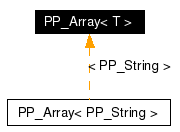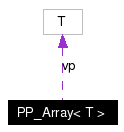# PP_Array< T > Class Template Reference

An Array of Objects of Type T for ParmParse. More...

`#include <ParmParse.H>`

Inheritance diagram for PP_Array< T >:[legend]
Collaboration diagram for PP_Array< T >:[legend]
List of all members.

## Public Member Functions

PP_Array ()
: Constructs an `PP_Array<t>' with no elements
PP_Array (long len)
PP_Array (long len, const T &initialvalue)
PP_Array (const T *vec, long len)
PP_Array (const PP_Array< T > &rhs)
: The copy constructor.
PP_Array< T > & operator= (const PP_Array< T > &rhs)
~PP_Array ()
: Deletes the `PP_Array<t>' and all objects it contains.
void clear ()
: Returns true if this `PP_Array<t>' is not empty.
void reserve (long _truesize)
void shrinkWrap ()
void resize (long newlen)
void resize (long newlen, const T &initialvalue)
long length () const
: Return number of elements in the array.
long trueSize () const
T & operator[] (long K)
const T & operator[] (long K) const
: Same as above, except acts on const PP_Array's.
T & get (long i)
: Different syntax for operator[] (long i).
const T & get (long i) const
: Different syntax for const operator[] (long i).
T * dataPtr ()
const T * dataPtr () const
: Same as above for constant PP_Arrays.
void set (long i, const T &elem)
: Changes the i'th element of this `PP_Array<t>' to elem.
void swap (long i, long j)
: This function swaps the i'th and j'th element of the PP_Array.
bool operator== (const PP_Array< T > &rhs) const
: Test for equality.
bool operator!= (const PP_Array< T > &rhs) const
: Test for inequality.

long truesize
long nelem
T * vp

## Detailed Description

### template<class T> class PP_Array< T >

An Array of Objects of Type T for ParmParse.

This class implements an array of objects of the parameterized type T. In contrast with the predefined C++ array type, an `PP_Array<t>' object knows its length, can be dynamically resized, and provides automatic bounds checking. The bounds checking can be turned off by specifying the -DNDEBUG flag on the command line when compiling the BOXLIB library. The main reason for defining the PP_Array class is that it is used, either by composition or inheritance, to implement many of the other classes in the BOXLIB library.

The `PP_Array<t>' class works by storing copies of the objects it contains. If the objects are large, such as `FARRAYBOX's it is probably better to use the `PArray' class which is an array class that stores pointers to the objects (avoiding expensive copies). The `PP_Array<t>' class destructs all objects in the array when it is itself destructed. If this is not the desired action, the `PArray' class should be used.

In the PP_Array<T> class, there are two different concepts of size: the amount of space allocated, and the amount of space actually used. Obviously, the allocated space must be larger than the used space. We separate these two concepts to allow the user to optionally avoid memory allocation costs. Rather than construct and destroy a temporary PP_Array<T> many times, it may be less expensive to allocate it once with enough memory to handle all uses, and resize the PP_Array<T> for each particular use. See the member functions `reserve', `shrinkWrap', and `resize'.

Note that care must be taken when deriving classes from `PP_Array<t>'. It is a concrete class, not a polymorphic one.

This class does NOT provide an assignment operator for assigning an integer to an PP_Array<T>.

This is a convenience class for ParmParse and will not be in any way supported by anyone at ANAG.

## Constructor & Destructor Documentation

 template PP_Array< T >::PP_Array ( ) ` [inline]`
 : Constructs an `PP_Array' with no elements

 template PP_Array< T >::PP_Array ( long len ) ` [inline]`
 : Constructs an `PP_Array' of length len with the value of each element defined by the default constructor for `T'.

 template PP_Array< T >::PP_Array ( long len, const T & initialvalue )
 : Constructs an `PP_Array' of length len with the value of each elements given by initialvalue.

 template PP_Array< T >::PP_Array ( const T * vec, long len )
 : Constructs an `PP_Array' of length len in which the K'th value is a copy of vec[K].

 template PP_Array< T >::PP_Array ( const PP_Array< T > & rhs )
 : The copy constructor.

 template PP_Array< T >::~PP_Array ( ) ` [inline]`
 : Deletes the `PP_Array' and all objects it contains.

## Member Function Documentation

 template void PP_Array< T >::clear ( ) ` [inline]`
 : Destructs each element in this `PP_Array'. The resulting array has length zero.

 template const T * PP_Array< T >::dataPtr ( ) const` [inline]`
 : Same as above for constant PP_Arrays.

 template T * PP_Array< T >::dataPtr ( ) ` [inline]`
 : Returns pointer to vector of data. This function breaks object encapsulation and should only be used for interfacing to Fortran subroutines.

 template const T & PP_Array< T >::get ( long i ) const` [inline]`
 : Different syntax for const operator[] (long i).

 template T & PP_Array< T >::get ( long i ) ` [inline]`
 : Different syntax for operator[] (long i).

 template long PP_Array< T >::length ( ) const` [inline]`
 : Return number of elements in the array.

 template bool PP_Array< T >::operator!= ( const PP_Array< T > & rhs ) const` [inline]`
 : Test for inequality.

 template PP_Array< T > & PP_Array< T >::operator= ( const PP_Array< T > & rhs )
 : This operator deletes the current `PP_Array' and replaces it with a copy of rhs.

 template bool PP_Array< T >::operator== ( const PP_Array< T > & rhs ) const
 : Test for equality.

 template const T & PP_Array< T >::operator[] ( long K ) const` [inline]`
 : Same as above, except acts on const PP_Array's.

 template T & PP_Array< T >::operator[] ( long K ) ` [inline]`
 : Returns a reference to the K'th element in this `PP_Array'. The element can be modified through this reference. The result can be used as an L-value.

 template bool PP_Array< T >::ready ( ) const` [inline]`
 : Returns true if this `PP_Array' is not empty.

 template void PP_Array< T >::reserve ( long _truesize )
 : Reserve space for future expansion of memory. You still must `resize' to address the memory.

 template void PP_Array< T >::resize ( long newlen, const T & initialvalue ) ` [inline]`
 : This function changes the size of this `PP_Array' to newlen while preserving the value of as many array elements as possible. If newlen is greater than the current length, the array is grown and the new elements have the value given by initialvalue. If newlen is less than the current length the array is cropped with the remaining elements retaining their original values.

 template void PP_Array< T >::resize ( long newlen ) ` [inline]`
 : This function changes the size of this `PP_Array' to newlen while preserving the value of as many array elements as possible. If newlen is greater than the current length, the array is grown and the new elements have the value given by the default constructor for `T'. If newlen is less than the current length the array is cropped with the remaining elements retaining their original values.

 template void PP_Array< T >::set ( long i, const T & elem ) ` [inline]`
 : Changes the i'th element of this `PP_Array' to elem.

 template void PP_Array< T >::shrinkWrap ( )
 : Shrink allocated memory to be just enough for elements in PP_Array. This is useful if you allocated a lot of memory to avoid memory allocation delays as you add elements. Once all the elements are added, you can reduce memory usage to the minimum by calling this function.

 template void PP_Array< T >::swap ( long i, long j ) ` [inline]`
 : This function swaps the i'th and j'th element of the PP_Array.

 template long PP_Array< T >::trueSize ( ) const` [inline]`
 : Return the maximum number of elements the array can hold without doing a `resize'.

## Member Data Documentation

 template long PP_Array< T >::nelem` [protected]`

 template long PP_Array< T >::truesize` [protected]`

 template T* PP_Array< T >::vp` [protected]`

The documentation for this class was generated from the following file:
Generated on Wed Oct 5 14:01:51 2005 for Chombo&AMRSelfGravity by1.4.1